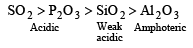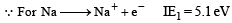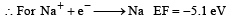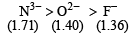Courses

# Test: 35 Year JEE Previous Year Questions: Classification of Elements & Periodicity in Properties

## 15 Questions MCQ Test Class 11 Chemistry 35 Years JEE Mains &Advance Past yr Paper | Test: 35 Year JEE Previous Year Questions: Classification of Elements & Periodicity in Properties

Description
This mock test of Test: 35 Year JEE Previous Year Questions: Classification of Elements & Periodicity in Properties for JEE helps you for every JEE entrance exam. This contains 15 Multiple Choice Questions for JEE Test: 35 Year JEE Previous Year Questions: Classification of Elements & Periodicity in Properties (mcq) to study with solutions a complete question bank. The solved questions answers in this Test: 35 Year JEE Previous Year Questions: Classification of Elements & Periodicity in Properties quiz give you a good mix of easy questions and tough questions. JEE students definitely take this Test: 35 Year JEE Previous Year Questions: Classification of Elements & Periodicity in Properties exercise for a better result in the exam. You can find other Test: 35 Year JEE Previous Year Questions: Classification of Elements & Periodicity in Properties extra questions, long questions & short questions for JEE on EduRev as well by searching above.
QUESTION: 1

### According to the Periodic Law of elements, the variation in properties of elements is related to their  

Solution:

According to modern periodic law, the properties of the elements are repeated after certain regular intervals when these elements are arranged in order of their increasing atomic numbers.

QUESTION: 2

### Which one of the following is an amphoteric oxide ?

Solution:

Na2O (basic), SO2 and B2O3 (acidic) and Zn O is amphoteric.

QUESTION: 3

### Which one of the following ions has the highest value of ionic radius ? 

Solution:

O– – and F are isoelectronic. Hence have same number of shells, therefore greater the nuclear charge smaller will be the size i.e.
O– – > F
further Li+ and B3+ are isoelectronic. therefore Li+  >  B3+ Hence the correct order of atomic size is.
O-- > F > Li+ > B3+

QUESTION: 4

Among Al2O3, SiO2, P2O3 and SO2 the correct order of acid strength is 

Solution:

As the size increases the basic nature of oxides changes to acidic nature i.e., acidic nature increases.SO2 and P2O3 are acidic as their corresponding acids H2SO3 and H3PO3 are strong acids.

QUESTION: 5

The formation of the oxide i on O2-(g) requires first an exothermic and then an endothermic step as shown below

O(g) + e- = O-(g) ΔHº =-142 kJmol-1                
O-(g) + e- = O2-(g) ΔHº= 844 kJmol-1
This  is because

Solution:

O ion exerts a force of repulsion on the incoming electron. The energy is required to overcome it.

QUESTION: 6

Which of the following oxides is amphoteric in character?

Solution:

CaO is basic as it form strong base Ca(OH)2 on reaction with water.
CaO + H2O –––––→ Ca(OH)2 CO2 is acidic as it dissolve in water forming unstable carbonic acid.
H2O + CO2 –––––→ H2CO3
Silica (SiO2) is insoluble in water and acts as a  very weak acid.
SnO2 is amphoteric as it reacts with both acid and base.
SnO2 + 2H2SO4–––––→ Sn(SO4)2 + 2H2O SnO2 + 2KOH–––––→ K2SnO3 + H2O

QUESTION: 7

In which of the following arrangements, the order is NOT according to the property indicated against it?

Solution:

In a period the value of ionisation potential increases from left to right with breaks where the atoms have some what stable configuration. In this case N has half filled stable orbitals. Hence has highest ionisation energy. Thus the correct order is
B < C < O < N
and not as given in option (c)

QUESTION: 8

Following statements regarding the periodic trends of chemical reactivity of the alkali metals and the halogens are given. Which of these statements gives the correct picture?

Solution:

The alkali metals are highly reactive because their first ionisation potential is very low and hence they have great tendency to loses electron to form unipositive ion.
NOTE : On moving down group- I from Li to Cs ionisation enthalpy decreases hence the reactivity increases. The halogens are most reactive elements due to their low bond dissociation energy, high electron affinity and high enthalpy of hydration of halide ion.
However their reactivity decreases with increase in atomic number

QUESTION: 9

In Which of the following arrangement, the given sequence is not strictly according to the property indicated against it ?

Solution:

(a,c) As we move from top to bottom in a group, the acidic strength of hydrides increases. Therefore, order of acidic strength of hydrides of VA and VII A group elements is

NH3 < PH3 < AsH3< SbH3
HF<HCl < HBr <HI

b) As we move from top to bottom in a group acidic nature (Ka) increases. Therefore, pKa decreases. Thus, order of pKa value of hydrides of VI A group  elements is
H2O > H2S >H2Se > H2Te

d) On moving from top to bottom, oxidising power of oxides increases. Thus, order of oxidising power of oxides of IVA group elements is
CO2 < SiO< SnO2 < PbO2

(a,c) As we move from top to bottom in a group, the acidic strength of hydrides increases. Therefore, order of acidic strength of hydrides of VA and VII A group elements is

NH3 < PH3 < AsH3< SbH3
HF<HCl < HBr <HI

b) As we move from top to bottom in a group acidic nature (Ka) increases. Therefore, pKa decreases. Thus, order of pKa value of hydrides of VI A group  elements is
H2O > H2S >H2Se > H2Te

d) On moving from top to bottom, oxidising power of oxides increases. Thus, order of oxidising power of oxides of IVA group elements is
CO2 < SiO< SnO2 < PbO2

QUESTION: 10

The correct sequence which shows decreasing order of the ionic radii of the elements is 

Solution:

A ll the given species con tain s 10 e each i . e. isoelectronic.
For isoelectronic species anion having high negative charge is largest  in size and the cation having high positive charge is smallest.

QUESTION: 11

Which one of the following orders presen ts the correct sequence of the increasing basic nature of the given oxides? 

Solution:

On moving across a period ionisation energy increases hence the electropositive nature of metals decreases therefore the ease of formation of ion also decreases and hence the basic character decreases. Further basic character of alkali metals oxides increases from Li2O to Cs2O. Hence the correct order will be Al2O3 < MgO < Na2O < K2O

QUESTION: 12

The increasing order of the ionic radii of th e given isoelectronic species is : 

Solution:

Among isoelectronic species ionic radii increases as the charge increases.
Order of ionic radii Ca2+ < K+ < Cl < S2– The number of electrons remains the same but nuclear charge increases with increase in the atomic number causing decrease in size.

QUESTION: 13

Wh ich of the following represents the corr ect order of increasing first ionization enthalpy for Ca, Ba, S, Se and Ar ? [JEE M 2013]

Solution:

On moving along a period from left to r ight I.E. increases and on moving down a group I.E. decreases. hence correct order is : Ba < Ca < Se < S < Ar

QUESTION: 14

The first ionisation potential of Na is 5.1 eV. The value of electron gain enthalpy of Na+ will be : [JEE M 2013]

Solution:(because the reaction is reverse)

QUESTION: 15

The ionic radii (in Å) of N3–, O2– and F are respectively : [JEE M 2015]

Solution:

For isoelectronic species, size of anion increases as negative charge increases. Thus the correct order is